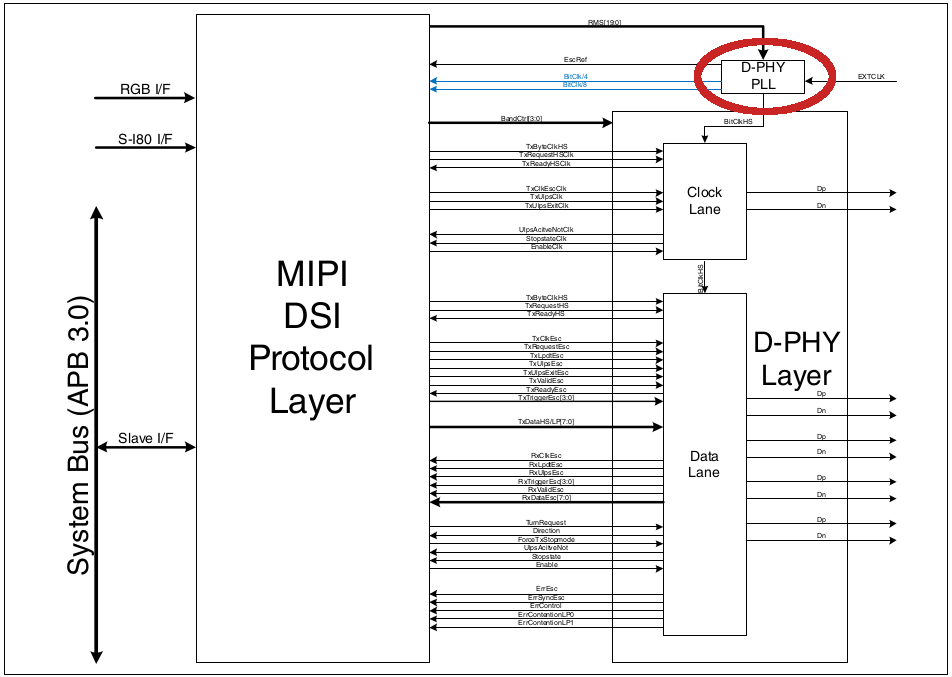# Setting i.MX8M Mini and Nano MIPI-DPHY Clock

cancel
Showing results for
Did you mean:

# Setting i.MX8M Mini and Nano MIPI-DPHY Clock

No ratings

## Setting i.MX8M Mini and Nano MIPI-DPHY Clock

The D-PHY PLL (in the red circle in the picture below) is the PLL that drives the MIPI Clock lane. It must be set in accordance with the video to be sent to the display.### Calculating the video bandwidth

The video bandwidth is calculated with the following equation:

Pixels per second = Horizontal res. x Vertical res. x Frame rate x Bits per pixel

Taking as example the 1080p60 OLED display RM67191:

Pixels per second = 1920 x 1080 x 60 x 24

Pixels per second = 2985984000 = 2,98Gpixels/sec

### Pixel clock calculation

The Display pixel clock can be obtained on the display driver. In this example for RM67191, the pixel clock is 132Mpixel/sec, see file:

Line 530:

.pixelclock = { 66000000, 132000000, 132000000 },

Or the number can be obtained with the following equation:

pixel clock = (hactive + hfront_porch + hsync_len + hback_porch) x (vactive + vfront_porch + vsync_len + vback_porch) x frame rate

pixel clock = (1080 + 20 + 2 +34) × (1920 + 10 + 2 + 4) x 60

pixel clock = 132000000 (rounded up)

### Bit clock calculation (clock lane)

The mipi-dphy bit_clk is the output clock and is calculated on file sec-dsim.c (line 1283):

Bit clock can be calculated with the following equation:

bit_clk = Pixel clock * Bits per pixel / Number of lanes

In the case of 1980p60 (Raydium display), It is:

bit_clk = pixel clock * bits per pixel / number of lanes

bit_clk = 132000000 * 24 / 4

bit_clk = 792000000

Other important timing parameters like 'p', 'm', 's' are obtained on the table in the following header file:

Labels (3)

• ### Linux

Version history
Revision #:
1 of 1
Last update:
‎12-20-2019 12:34 PM
Updated by: